# Java应用OpenvCV指南其六：利用OpenCV实现的数字识别（验证码识别）

之前介绍了很多概念上的东西，这次让我们来进行一次实际的应用。数字识别可以应用在许多领域，如数字型验证码的识别，车牌识别等领域。下面借我之前完成的一个数字验证码识别的小项目来简单认识一下图象识别领域的知识。

如果在阅读这篇文章时有什么疑问，可以参考一下之前的一些文章：

1、图象预处理
图像预处理，是将待检测图像交给识别模块识别。在图像分析中，对输入图像进行特征提取、分割和匹配前所进行的必要处理。
图像预处理的主要目的是消除图像中无关的信息，恢复有用的真实信息，增强有关信息的可检测性和最大限度地简化数据，从而提高特征抽取、图像分割、匹配和识别的可靠性。
在识别数字的时候，预处理的最终目标一般都是生成一张原图的清晰二值化图象，所谓二值化就是将要识别的图像变成只有黑白两种颜色的单通道图片。经过这样处理后的图像特征突出，颜色简单，可以大大的降低我们识别时的难度和复杂度。

Imgproc.threshold(src, dst, thresh, maxval, type);

• 第一个参数，Mat型的src为待处理的原图。
• 第二个参数，Mat型的dst为输出的图象。
• 第三个参数为阈值。
• 第四个参数表示最大值。
• 第五个参数表示二值运算的方式，参数数值说明如下：
enum
{
CV_THRESH_BINARY =0, /* value = value > threshold ? max_value : 0 */
CV_THRESH_BINARY_INV =1, /* value = value > threshold ? 0 : max_value */
CV_THRESH_TRUNC =2, /* value = value > threshold ? threshold : value */
CV_THRESH_TOZERO =3, /* value = value > threshold ? value : 0 */
CV_THRESH_TOZERO_INV =4, /* value = value > threshold ? 0 : value */
CV_THRESH_OTSU =8 /* use Otsu algorithm to choose the optimal threshold value; combine the flag with one of the above CV_THRESH_* values */
};

http://www.opencv.org.cn/opencvdoc/2.3.2/html/doc/tutorials/imgproc/threshold/threshold.html

不过相对于识别需要的内容，我们获得的原图一般都包含过多的图象信息，直接做二值化的话很难调参，即使勉强操作得出的图象也几乎无法进行识别，所以我们再二值化之前需要预先做一些处理尽量去除干扰要素。特别是验证码图象，一般都会有一些干扰元素在里面，很多情况下需要具体问题具体分析。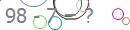图上有许多噪点和弧形的彩色干扰线条，我在预处理时先写了一个算法尽可能的消除了这些干扰线，然后做了滤波，最后得出了类似这样的效果：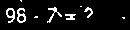这样的话差不多就满足识别的要求了，处理时注意方法的通用性，因为验证码是随机生成的，所以我们需要保证处理后大多数的图象效果都在一个水平线上，以保证我们识别的准确率。

2、图象的切割（选定ROI区域）
获得二值化的图象以后需要对图中的数字进行切割，分割成一个个单独的数字，由于二值化后 每个像素点的值只有0和maxval两种（单通道），还是以上图为例，图中黑色为0，白色为255。

Rect rect=new Rect(x1,y1,x2,y2);
Mat Img1 =New Mat(img , rect）;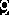3、制作模板

4、数字识别

Core.absdiff(src1, src2, dst);

• 第一个参数，src1第一个原数组。
• 第二个参数，src2第二个原数组。
• 第三个参数，dst输出数组。

dst(I)c = abs(src1(I)c - src2(I)c).

所有数组必须有相同的数据类型相同的大小（或ROI大小）

1、读取图片，并对干扰线进行初步处理

        Mat img=Imgcodecs.imread("F:/workspace/opencv/train/29.jpg");
Mat end=new Mat();

//去除干扰线

for(x=0;x<img.rows();x++){
for(y=0;y<img.cols();y++){
double[] clone=img.get(x, y).clone();   //修改通道数值
double cb=clone;
double cg=clone;
double cr=clone;
double avg=(cb+cg+cr)/3;
if(!((cb>c&&cg>c&&cr>c)&&((avg>98)&&(avg<148)||(avg>153)&&(avg<196))&&((max(clone)-min(clone))<55))){
clone=255;
clone=254;
clone=255;
img.put(x, y, clone);
}
}
}

Imgproc.bilateralFilter(img, end, a, a*2, a/2);  //双边滤波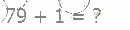2、转换为灰度图，并用中值滤波对图象进行进一步处理，最后将预处理过的图象二值化。

        Mat end2=new Mat();
Imgproc.cvtColor(end, end2, Imgproc.COLOR_BGR2GRAY);  //灰度图
Imgproc.medianBlur(end2, end2, 3);  //中值滤波
Imgproc.threshold(end2, end2, 245, 255, 1);  //二值化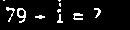3、通过扫描每列与每行点的值，截取图片上的数字

        Rect area1=new Rect(x1,y1,a1,b1);
Rect area2=new Rect(x2,y2,a2,b2);
Rect area3=new Rect(x3,y3,a3,b3);

Mat num1=new Mat(end2,area1);
Mat num2=new Mat(end2,area2);
Mat num3=new Mat(end2,area3);

Rect的值为要截取部分左上角和右下角的坐标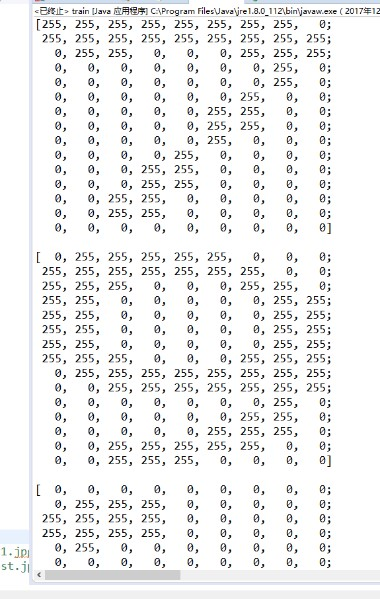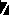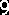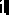4、通过截取的数字制作标准模板
纯手工制作，不过步骤比较繁琐单调。具体来说就是从截取的数字中挑选读取比较标准的数字，二值化后反复通过get.clone()和put方法修改相应像素点的颜色具体操作方式参考上面去除干扰线的方法，这里就不再说明了。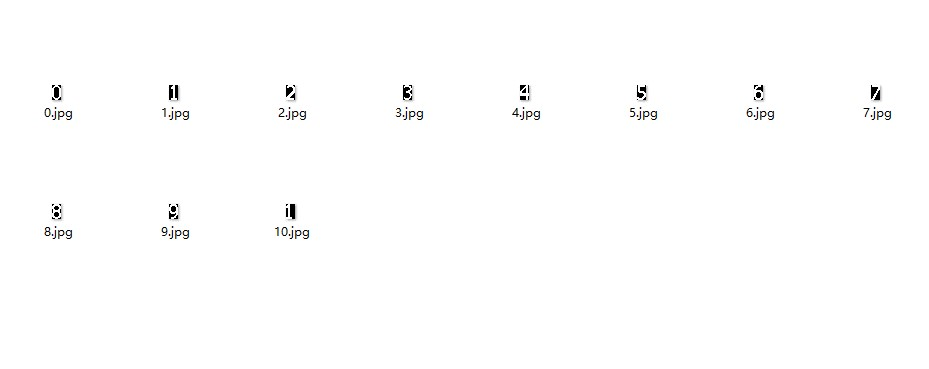5、数字识别

    public static int getnum(Mat number){
int num=0;
int min=30000;
Mat model=new Mat();
Mat mid=new Mat();
Mat res=new Mat();

for(int i=0;i<=10;i++){
Imgproc.cvtColor(model, mid, Imgproc.COLOR_BGR2GRAY);
Imgproc.threshold(mid, mid, 250, 255, 0);  //二值化
Core.absdiff(number, mid, res);  //两个矩阵的差的绝对值
int now=getsum(res);
if(now<min){
min=now;
num=i;
}
}
if(num==10)
num=1;

return num;
}

        boolean pls=false;
for(int i=45;i<130;i++){
for(int j=6;j<24;j++){
double[] can=end2.get(j, i).clone();
double s=can;
sum+=s;
}
if (sum>=510){
p=i;
break;
}
sum=0;
}
if(p>=50)
pls=true;

6、输出结果通过模拟登录的方式请求登录页面验证码，然后下载图片识别的方式测试实例80次，成功登录79次，识别率为98.75%©️2019 CSDN 皮肤主题: 编程工作室 设计师: CSDN官方博客